#.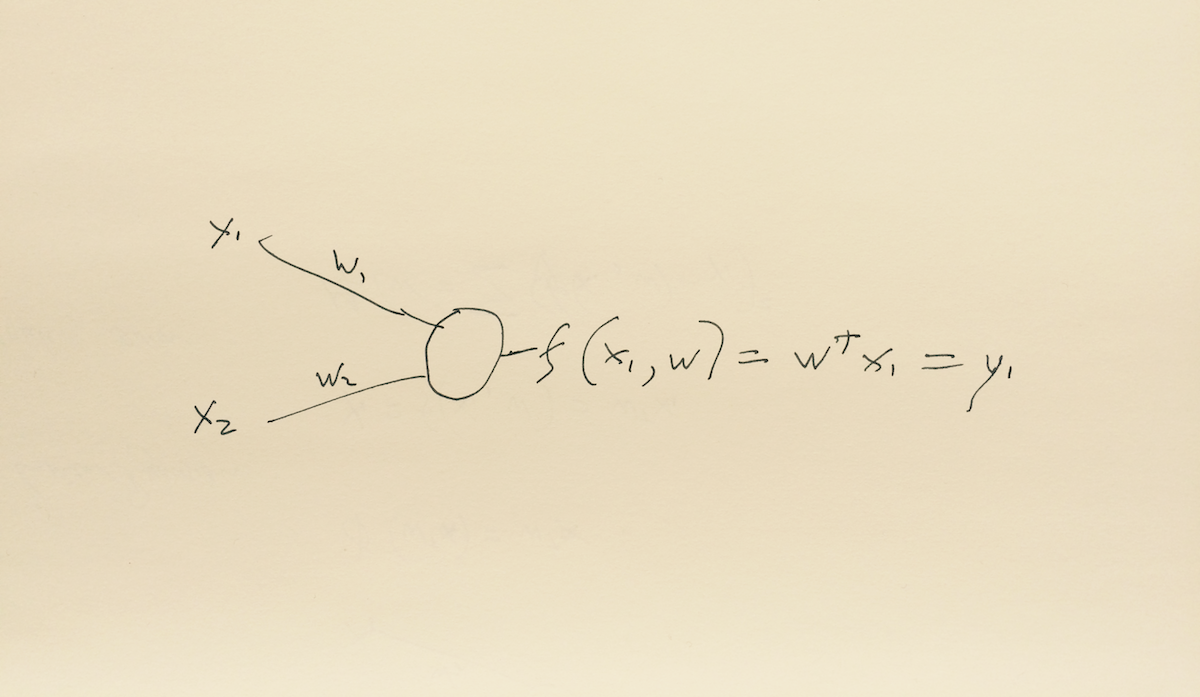Machine learning is a loose term, it covers many activities. From a software engineering aspect it could be seen as an activity evolved from pattern recognition. It can be even more narrowly viewed as the assignment of a label to a given input value. A system designed to learn from examples would contain a classifier that takes data as input and assigns a label to it as output.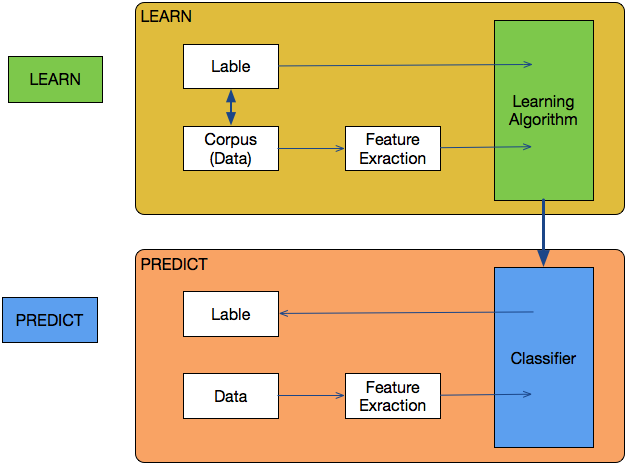```X = np.array([[1,2],[5,8],[1.5,1.8],[8,8],[1,0.6],[9,11]])y = [0,1,0,1,0,1]
clf = svm.SVC(kernel='linear', C = 1.0)
clf.fit(X,y)
print(clf.predict([0.58,0.76]))```

The above is the source code for a machine learning program written in python using the SciKit-Learn library. Not that much to it really! Below is the python function to calculate the square root of a number.

`math.sqrt( x )`

Like all toolsets SciKit-learn gives developers, programmers and data analysts a combination of functionality. It is a comprehensive machine learning technology aimed at data mining and data analysis. It works well on its own. It works well with other technologies such a Tensor Flow. It works well within other programs.

As developers we often use many tools, an example shown above is the Math function built into python. We can use the tool to perform maths without knowing how it works. The example I give of calculating a square root of a number is more complex than we think, but we often take it for granted, and this does not stop us from using it competently. There are many ways to skin a cat and there are many ways that computer programs and calculators find the square root of a number.

It does help when developing software to know the bare bones of the technological tools that you use. To see the innards behind a functionality, to know the relationship between inputs and outputs, to have a picture of the what is going on. I can understand these complications by viewing, snippets of code, diagrams, formula and maths. This post, while technical in nature, skims across the surface of Machine learning in a flighty manner. Fuller explanations are available, I recommend three:

### WHY EMPLOY MACHINE LEARNING?

There are things that computer programs do very well that are difficult for humans. From simple calculations such as squaring a number;

```static void main(String[] args) {      int x = 5;
x = x*x;
System.out.println("x = " + x);
}
}
x = 25```

… to slightly harder calculations;

```public class Main {   public static void main(String[] args) {
double x = 38.75645;
x = x*x;
System.out.println("x = " + x);
}
}
x = 1502.0624166025```

… to more complex processes such as finding the square of a number.

While squaring a number is simple, the problem of finding a square root of a number involves more convoluted maths.

As the square root of S can be represented by the equation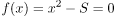there are a number of algorithms that can be used to find the answer. The Babylonian method discovered by Newton is an example;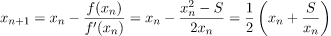It is much easier to call a programmatic function such as the Math function in Javascript;

`var y = Math.sqrt(16);      // Returns the square root of 16, y = 4`

Programing languages allow computers to do things like calculations and maths very well. However there are things that humans do well that computers are not so good at. An example of something computers have not done so well could be making a decision based on disparate inputs or understanding speech.

Machines struggle to interpret speech and text as we naturally speak and type it. Artificial neural networks (ANN) are one way to overcome this problem. To understand how they can overcome the problem it is useful to look at how an ANN differs from a conventional computer program.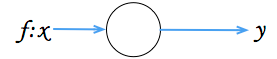A conventional program works by using a central processor, reading an instruction and using data stored at a specific address to follow predetermined procedures. It does this regardless of it being written in a; procedural, object oriented or any other type of language.

Running a single bit of code, for example a single function, delivers a single output. Programing languages tend to run one process and then move on to the next. The outcome of one function results in another function being called. While some programs allow parallel processes to be run simultaneously and repeat processes iteratively in the main there is a logic flow and one thing follows another.

Most programs follow quite complex instructions and are made up of many interlinked sub routines, they follow a logical flow that could have many paths. Every fork in the road and every path has to be explicitly written and complex decisions require complex instructions.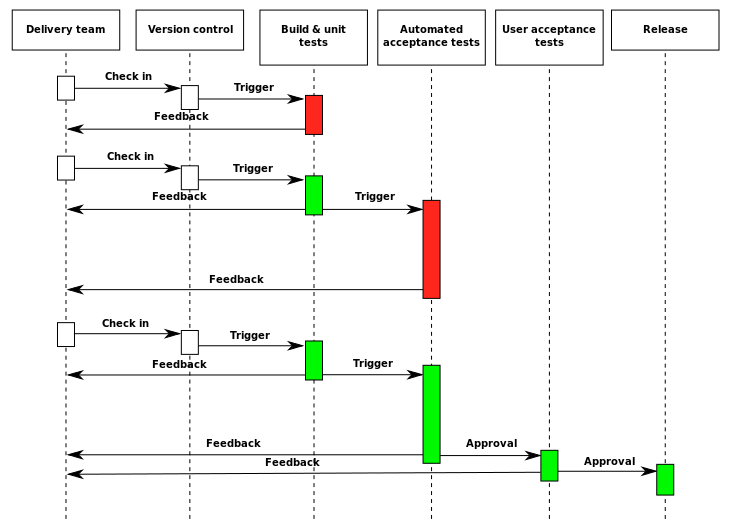The UML CI

Neural networks work in a different way from most current computer programs. They do not process a complex set of programmatic instructions by running a branching system of code using a central processor. Instead they carry out a large number of very simple programs, often across a large number of processors, using the weighted sums of their inputs.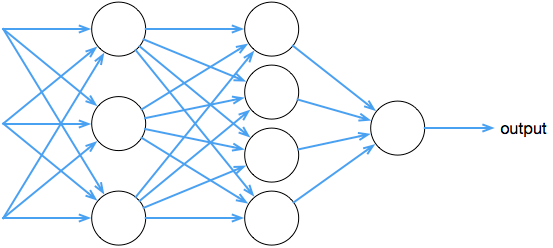A network of these simple programs provides a result that can accommodate subtle changes and respond to less definite bits of information. The network can generalise and find patterns that may be hidden. In this sense, it works in a similar way to the biological model that it is named after.

### THE COMPUTATIONAL PROCESS

#### PERCEPTRON

To start to illustrate the computational process we will look at a very simple example of a neural network. First invented 60 odd years ago it is a perceptron. It is a “feed-forward” model; inputs are sent into the neuron, processed, and then output. The perceptron starts by calculating a weighted sum of its inputs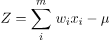The perceptron has five parts:

1. Inputs: X1, X2
2. Weights: W1 W2
3. Potential: where
4. Activation function: f(z)
5. Output: y = f(z)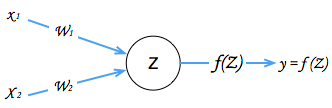We can ask the perceptron to give us an answer to a question where we have three factors that influence the outcome. For example “Is this good food?”. The factors that make it good or bad are;

“is it good for you?”
“does it taste good?”
“does it look good?”

We give numerical values to all of the questions and the answers. We give each question a value, in this case a boolean, yes or no, 1 or 0. We give the same values to the answer, good food = 1, not good food =-1.

We collect some data, and convert the values it into numbers .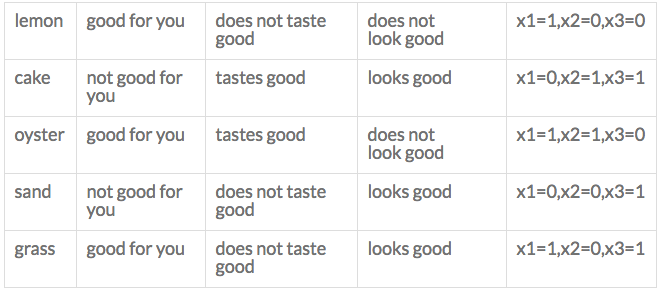Now we assume that if it is “good for you” is most important factor but the taste and the appearance will also influence the answer. We consider how important each factor has and give it a weight accordingly.

Lets pass in three inputs to our perceptron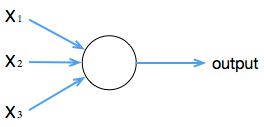```Input_0: x1 = 0Input_1: x2 = 1
Input_2: x3 = 1```

The next thing we have to do is give some weights to these questions, we assume they are not all equal in importance. We guess how important they are.

```Weight_0: x1 = 6Weight_1: x2 = 4
Weight_2: x3 = 2```

the inputs are multiplied by the weights

```Input_0 * weight 0: 0 * 6 = 0Input_1 * weight 1: 1 * 4 = 4
Input_1 * weight 1: 1 * 2 = 2```

The next step is to sum all the inputs and the weights

`output = sum(0+4+2) = 6`

The neuron’s output is determined by whether the weighted sum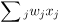is less than or greater than a thresholdValue. Just like the weights, the threshold is a real number which is a parameter of the neuron.

The following is the equation used to calculate the outputs of the neuron;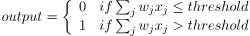```int threshold = 7
int activate(sum) {
if (sum => threshold) return 1;
else return -1;
}```

So adding all the input values and the weights the our single cell perceptron tells us it what “is good food”. As we know the answers to the question we can use the answers to adjust

Now all this is very basic and it would be easy to write a few lines of code to work out that we have three conditions that have a value and are weighted, measure the output against our threshold it can then make a decision of true or false.

The power of ANNs comes from having very many neurones networked together. Adding more inputs and using more layers we can add subtle values to the factors that influence the decision. Better still we can get the network to learn to adjust these factors.

### ARTIFICIAL NEURAL NETWORK (ANN)

#### TRAINING THE NETWORK USING LINEAR REGRESSION.

A single layer, single neuron network (using a linear activation function) receives an input with two features x1 and x2; each has a weight. The network sums the inputs and weights, then outputs a prediction. The difference is calculated to measure the error showing how well it performs over all of the training data.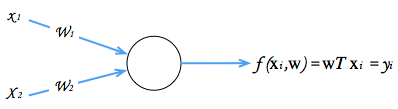```var forwardF = function(x, y) {  return x * y;
};
forwardF(-2, 3); // returns -6```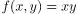We use a network to change the output as we want a number that is slightly bigger than -6. We move forward through the network guessing what values for x and y would give us a good fit.

```var forwardF = function(x, y) { return x * y; };var x = -2, y = 3;

var tweak_amount = 0.01;
var best_out = -Infinity;
var best_x = x, best_y = y;
for(var k = 0; k < 100; k++)
{ var x_try = x + tweak_amount * (Math.random() * 2 - 1);
var y_try = y + tweak_amount * (Math.random() * 2 - 1);
var out = forwardMultiplyGate(x_try, y_try); if(out > best_out) {
best_out = out;
best_x = x_try, best_y = y_try;
}
}```

This works well when we are seeking to answer a few questions asked of a small amount of data.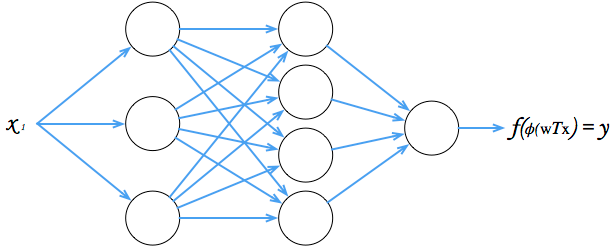Instead of simply adjusting the weight of a single input we could look at a derivative of the output to change two or multiple inputs. By using a derivative we could use the output to lower one input and increase the other. A mathematical representation of the derivative, could be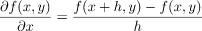```var x = -2, y = 3;var out = forwardF(x, y); // -6
var h = 0.0001;

// compute derivative with respect to x
var xph = x + h; // -1.9999
var out2 = forwardMultiplyGate(xph, y); // -5.9997
var x_derivative = (out2 - out) / h; // 3.0

// compute derivative with respect to y
var yph = y + h; // 3.0001
var out3 = forwardMultiplyGate(x, yph); // -6.0002
var y_derivative = (out3 - out) / h; // -2.0```

#### ACTIVATION

An artificial neural network processes information collectively, in parallel across a network of neurons. Each neuron is in itself a simple machine. It reads an input, processes it, and generates an output. It does this by taking a set of weighted inputs, calculating their sum with a function to activate the neuron

and passing the output of the activation function to other nodes in the network.

As the activation functions takes two (same-length) sequences of numbers (vectors) to returns a single number. The operation can be expressed (algebraically) as.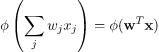Below is an expression of a linear activation function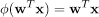A model of a system with a linear feature to produce a single output is expressed by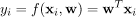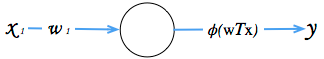A characteristic of a neural network is it’s ability to learn so they sit under the machine learning heading very comfortably. The network may form a complex system, that is agile and can adapt. It can modify its internal structure based on the information it is given. In other words it learns from what it is receives and from processing this as outputs. In artificial neural networks the classifier seeks to identify errors within the network and then adjust the network to reduce those errors.

In general terms a network learns from having an input and a known output, so we can give pairs of values (x, y) where x is the input and y the known output

```pairs = ((x=5,y=13),(x=1,y=3),(x=3,y=8)),...,((x=<em>n</em>,y=<em>n2</em>))
```

The aim is to find the weights (w) that fit closest to the training data. One way to measure our fit is to calculate the minimum error over the dataset by reducing the value of M(w) to a minimum.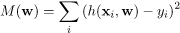First published on the XCiPI blog. In the next post in this series I will look at back propagation a bit more deeply and why classifying data by query functions can lead to a natural language interface that will revolutionise analytics and business intelligence.

Views: 2251

Comment